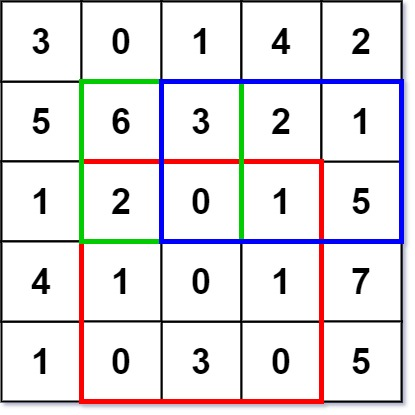# Range Sum Query 2D – Immutable LeetCode Solution

Difficulty Level Medium

## Problem Statement

Range Sum Query 2D – Immutable LeetCode Solution – Given a 2D matrix, handle multiple queries of the following type:

• Calculate the sum of the elements of the matrix inside the rectangle defined by its upper left corner (row1, col1) and lower right corner (row2, col2).

Implement the NumMatrix class:

• NumMatrix(int[][] matrix) Initializes the object with the integer matrix matrix.
• int sumRegion(int row1, intcol1, int row2, int col2) Returns the sum of the elements of the matrix inside the rectangle defined by its upper left corner (row1, col) and lower right corner (row2, col2).

Example 1:Input

```["NumMatrix", "sumRegion", "sumRegion", "sumRegion"]
[[[[3, 0, 1, 4, 2], [5, 6, 3, 2, 1], [1, 2, 0, 1, 5], [4, 1, 0, 1, 7], [1, 0, 3, 0, 5]]], [2, 1, 4, 3], [1, 1, 2, 2], [1, 2, 2, 4]]
```

Output

```[null, 8, 11, 12]

```

Explanation

```NumMatrix numMatrix = new NumMatrix([[3, 0, 1, 4, 2], [5, 6, 3, 2, 1], [1, 2, 0, 1, 5], [4, 1, 0, 1, 7], [1, 0, 3, 0, 5]]);
numMatrix.sumRegion(2, 1, 4, 3);  return 8 (i.e sum of the red rectangle)
numMatrix.sumRegion(1, 1, 2, 2);  return 11 (i.e sum of the green rectangle)
numMatrix.sumRegion(1, 2, 2, 4);  return 12 (i.e sum of the blue rectangle)```

## Approach

### Idea:

First, we make a cumulative sum for each row. Then, we use this new cumulative array and subtract the last int in our new “sumRegion” row from the first number in that “numMatrix” row. This shows us how much each row is and after we find the sums of each row, add them together to figure out the sum of the “sumRegion”. For regions that are taller than they are wide, do this same process but with the columns instead. The link down below shows the process written out.

## Code

### Python Program of Range Sum Query 2D – Immutable

```class NumMatrix :
dp = None
def __init__(self, matrix) :
self.dp = [ * (len(matrix)) for _ in range(len(matrix))]
self.populateArray(matrix, self.dp)
def populateArray(self, arr, dp) :
i = 0
while (i < len(arr)) :
j = 0
while (j < len(arr)) :
if (j == 0) :
dp[i][j] = arr[i][j]
else :
dp[i][j] = arr[i][j] + dp[i][j - 1]
j += 1
i += 1
def sumRegion(self, row1, col1, row2, col2) :
if (row2 >= len(self.dp) or col2 >= len(self.dp) or row1 < 0 or col1 < 0) :
return -1
sum = 0
i = row1
while (i <= row2) :
sum = sum + (self.dp[i][col2] - (self.dp[i][col1 - 1] if col1 > 0 else 0))
i += 1
return sum```

### Java Program of Range Sum Query 2D – Immutable

```class NumMatrix {

int[][] dp;

public NumMatrix(int[][] matrix) {
dp = new int[matrix.length][matrix.length];
populateArray(matrix, dp);
}

private void populateArray(int[][] arr, int[][] dp) {
for (int i = 0; i < arr.length; i++) {
for (int j = 0; j < arr.length; j++) {
if (j == 0) {
dp[i][j] = arr[i][j];
} else {
dp[i][j] = arr[i][j] + dp[i][j - 1];
}
}
}
}

public int sumRegion(int row1, int col1, int row2, int col2) {
if (row2 >= dp.length || col2 >= dp.length || row1 < 0 || col1 < 0)
return -1;
int sum = 0;
for (int i = row1; i <= row2; i++) {
sum = sum + (dp[i][col2] - (col1 > 0 ? dp[i][col1 - 1] : 0));
}
return sum;
}
}```

## Complexity Analysis for Range Sum Query 2D – Immutable LeetCode Solution

### Time Complexity

The time complexity of the above code is O(n^2) because there is a nested for loop.

### Space Complexity

The space complexity of the above code is O(n) because we are using an array to store the prefix values of the original array.

Translate »